### Home > MC2 > Chapter 10 > Lesson 10.1.4 > Problem10-46

10-46.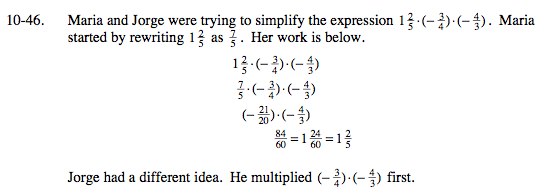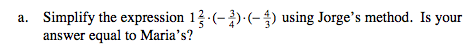$\text{What do you get when you multiply }\left(-\frac{3}{4}\right) \cdot \left(-\frac{4}{3}\right)?$

Since you are multiplying by reciprocal fractions, the fractions reduce to 1.

$\text{Yes, }1\frac{2}{5} \cdot \left(-\frac{3}{4}\right) \cdot \left(-\frac{4}{3}\right) = 1\frac{2}{5}$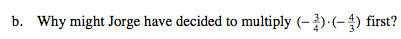$\text{As you found in part (a), }\left(-\frac{3}{4}\right)\left(-\frac{4}{3}\right) \text{ is 1, due to the multiplicative property.}$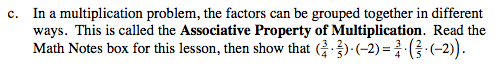Solve each equation. What do you get?
$-\frac{12}{20} = -\frac{12}{20}$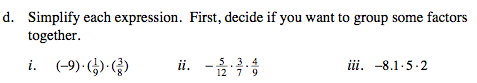$ii. -\frac{5}{60}$